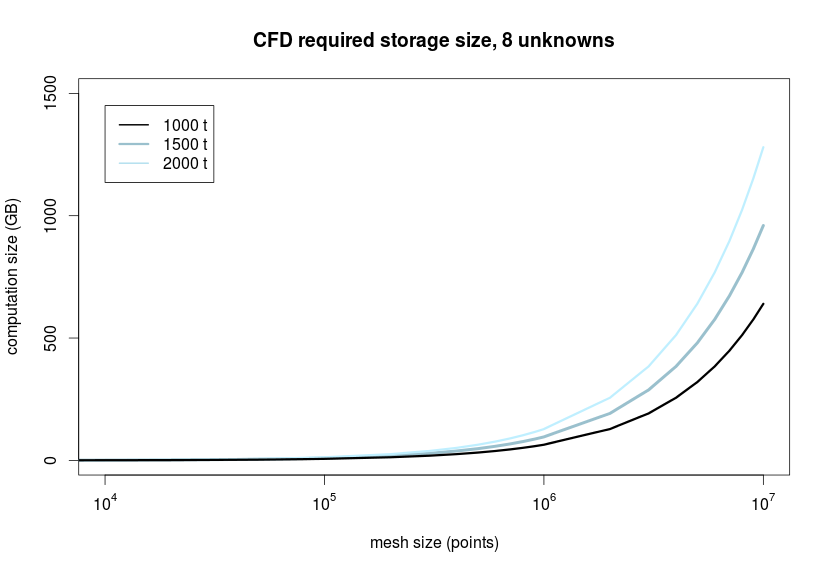# CFD file sizes

I’m presently taking a computational fluid dynamics (CFD) course here at Rice (taught by Dr. Tayfun E. Tezduyar), and I was shocked to learn the sheer volume of data generated from a typical CFD simulation, and the digital storage required to be able to look back at the modeled results. A homework problem was the following:

“Consider a 3D computation of air circulation in a room with temperature effects. There are also 3 chemical species (e.g. 3 pollutants) we want to keep track of. The species concentrations are so low that they do not influence the fluid density or velocity. The number of grid points is 10 million, and the simulation takes 1000 time-steps. Assuming that a number takes 8 bytes, how much disk storage do you need to store all the computed data?”

By my calculations, you would generate approximately 640 Gigabytes (GB) of data in the process of solving this problem!

In the figure below you can see how mesh size influences the storage size of the generated data for three different simulation lengths. I have isolated the figure to the interesting data (everything with a mesh size below 10^4 points generates relatively small data volumes). All model parameters are provided below the figure.Model parameters:

• 8 unknowns for every mesh point at every timestep
• conservation of momentum = 3
• conservation of energy = 1
• velocity = 1
• species to track = 3
• evenly spaced time-steps of 1000, 1500, and 2000
• 8 bytes are required to store a single unknown (meaning one value at one point, at one time)

Interesting note: increasing the complexity of your problem without increasing the number of unknowns has no effect on the storage size, but only on the time required to complete the simulation!

Updated: